### PRODUCT Function Examples in Excel, VBA, & Google Sheets

This Tutorial demonstrates how to use the Excel PRODUCT Function in Excel to multiply an array of numbers.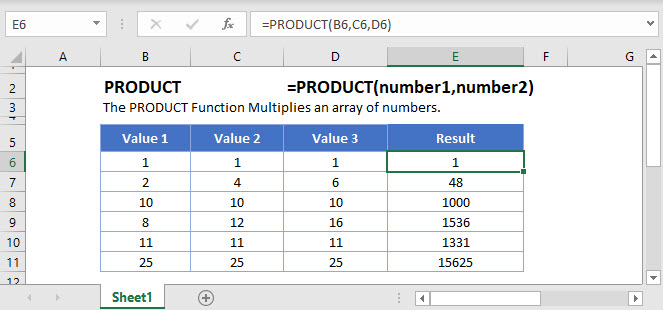## PRODUCT Function Overview

The PRODUCT Function Multiplies an array of numbers.

To use the PRODUCT  Excel Worksheet Function, select a cell and type: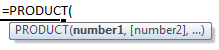(Notice how the formula inputs appear)

## PRODUCT Function Syntax and Inputs:

number1 – Two or more numbers, separated by commas, or an array of numbers.

AutoMacro - VBA Code Generator

## PRODUCT Function

The PRODUCT Function returns the product of the numbers entered in the function.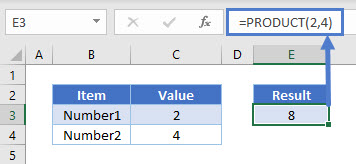## PRODUCT Function – Cell Reference

The PRODUCT Function returns the product when cell references are entered as argument.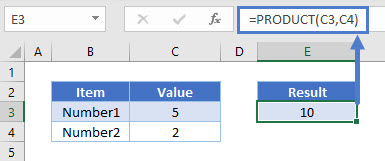Here cell C3 is multiplied by cell C4.

## PRODUCT Function – Cell Range

The PRODUCT Function can also return the product if a range is entered.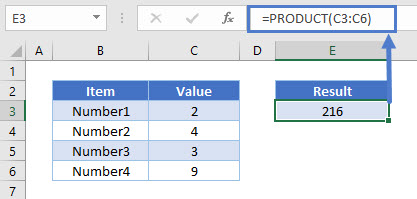The formula will multiply the cells in the range i.e. C3 * C4 * C5 * C6.

## PRODUCT Function – Array

The PRODUCT Function can return the product if an array is entered as an argument.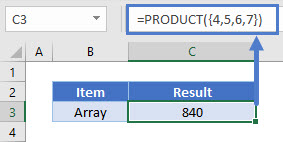The function will multiply 4, 5, 6, & 7.

## PRODUCT Function – Different Ranges

The PRODUCT Function can take two or three different ranges and return their product.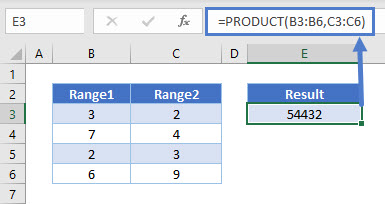Here the function will return the product of the following cells: B3, B4, B5, B6, C3, C4, C5, C6.

## PRODUCT Function – Empty Cell

The PRODUCT Function ignores empty cells when it returns the product of a given range.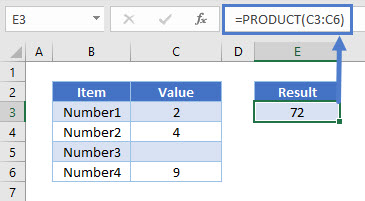## PRODUCT Function – Cell with Text

Likewise, the PRODUCT Function ignores any cell with text when it returns the product of a given range.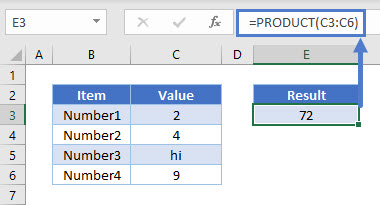### SUMPRODUCT

SUMPRODUCT Function returns of the sum of the product of corresponding members of an array or range.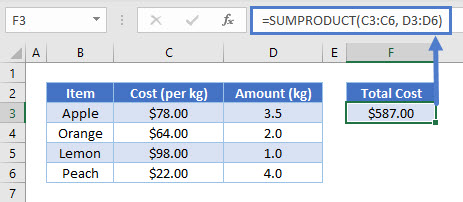Here the function multiples C3 * D3, C4 * D4, C5 * D5&  C6 * D6 and returns the sum of these products.

## PRODUCT in Google Sheets

The PRODUCT Function works exactly the same in Google Sheets as in Excel: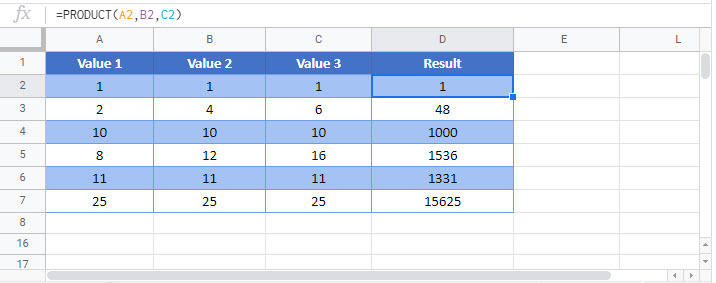## Additional Notes

Use the PRODUCT Function to multiply an array of numbers.
Instead of typing:
` =a1*a2*a3*a4`
you can enter:
`=product(a1:a4)`

## PRODUCT Examples in VBA

You can also use the PRODUCT function in VBA. Type:
`application.worksheetfunction.product(number1,number2)`
For the function arguments (number1, etc.), you can either enter them directly into the function, or define variables to use instead.

Return to the List of all Functions in Excel

## Excel Practice Worksheet

Practice Excel functions and formulas with our 100% free practice worksheets!

• Automatically Graded Exercises
• Learn Excel, Inside Excel!